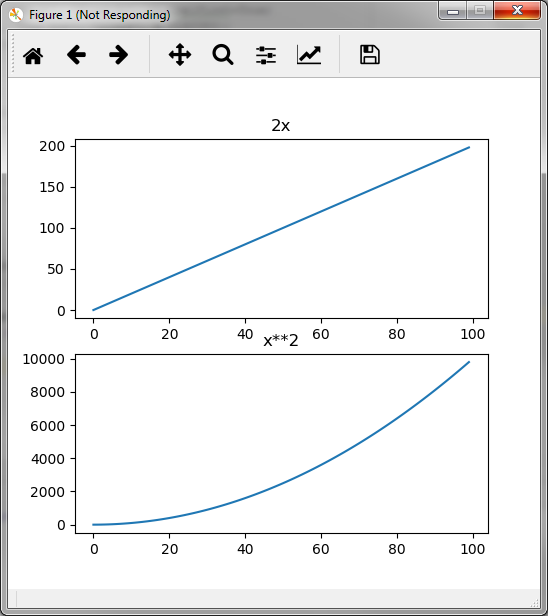# Plotting data¶

Often we want to plot loss or accuracy of a network training over time to get an impression of convergence speed. nuts-ml provides a simple plotting nut to facilitate this need. The following example demonstrates the basic usage by plotting a quadratic function

```  >>> samples = (x**2 for x in range(100))
>>> samples >> PlotLines(None) >> Consume()

.. image:: pics/plot_squares.png
```

Note that the plot updates live with each sample read from `samples` and closes immediately once the plotting is finished. Since the samples are just numbers we specify `None` as column index in `PlotLines`.

`PlotLines` allows to plot multiple sample columns. In this next example we plot ``2*x` and `x**2` and specify the corresponding sample columns `(0,1)` in `PlotLines`:

```>>> samples = ((2*x, x**2) for x in range(100))
>>> samples >> PlotLines((0,1), layout=(2,1), titles=('2x','x**2')) >> Consume()
```

We want the two plots of the figure to appear in one column (instead of next to each other) and therefore set the `layout` to 2 rows and 1 column. `titles` allows to set titles for the individual plots.Let us conclude this section with a more complex example. The following code sketch plots the loss and accuracy of a network training per batch and per epoch. The generated figures are written to image files in PNG format instead of being displayed:

```plot_batch = PlotLines((0,1), every_sec=5, filepath='batch.png')
plot_epoch = PlotLines((1,2), 0, layout=(2,1), filepath='epoch.png')
mean = Mean()

for epoch in range(EPOCHS):
t_loss, t_acc = (train_samples >> ... >> build_batch >>
network.train() >> plot_batch >> Unzip())
plot_epoch( (epoch, mean(t_loss), mean(t_acc) )
```

For a large train set many batches will be created and losses computed. We are only interested in a subset of those and set `every_sec=5` to plot batch-wise loss and accuracy every 5 seconds. Alternatively we could specify `every_n=10` to plot for every 10-th batch.

When plotting the mean loss and average per epoch via `plot_epoch` we provide a tuple of format `(<epoch>, <mean loss>, <mean acc>)`. We want the x-axis to show the epoch and the y-axes to show loss and accuracy. The column indices specified in `PlotLines` for the y-axes are therefore `(1,2)` and the values for the x-axis are in column 0 of the samples. Run `help(PlotLines)` for more details and the description of other parameters.